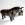### fx_lmcapStrategy2021 (ver. 3) Running \$(function () { DFC.StrategyStatus.user_id = 3108; \$('body').initStrategyStatusUpdater(190703); \$('#status-animation-190703').initStrategyStatusAnimation(); });The strategy trades GBPUSD based on a momentum indicator, the Relative Strength Index (RSI). Only one order per day is allowed. There are 2 types of orders: type A and type B. Orders type A can be opened from 6 AM to 15 PM and from Monday to Thursday; on Fridays, the schedule is different, from 6 AM to 12 AM. The trading period for orders type A is 1-hour, whilst for orders type B, the period is 10-sec. To open a buy order type A, the following conditions have to be verified: the RSI(current bar) equal or lower than 30; the RSI(previous bar) equal or lower than 30; spread equal or lower than 2 pips; and the difference between the daily high and the daily low equal or higher than 75 pips. To open a sell order type A, the following conditions have to be verified: the RSI(current bar) equal or higher than 70; the RSI(previous bar) equal or higher than 70; spread equal or lower than 2 pips; and the difference between the daily high and the daily low equal or higher than 75 pips. The orders type A have the following assignments: take profit @ 10 pips; stop loss @ -55 pips; and slippage @ 2 pips. If equity drops below 99.000, the take profit assignment of orders type A changes from 10 pips to 25 pips. The orders type B can be opened from 15 PM to 24 PM and from Monday to Thursday; on Fridays, the schedule is different, from 12 PM till the close of trading. To open a buy order type B, the following conditions have to be verified: the RSI(current bar) equal or lower than 30; the RSI(previous bar) equal or lower than 30; spread equal or lower than 2 pips; and the difference between the daily high and the daily low equal or higher than 45 pips. To open a sell order type B, the following conditions have to be verified: the RSI(current bar) equal or higher than 70; the RSI(previous bar) equal or higher than 70; spread equal or lower than 2 pips; and the difference between the daily high and the daily low equal or higher than 45 pips. The orders type B have the following assignments: take profit @ 1/2 of take profit assignment of orders type A; stop loss @ -55 pips; and slippage @ 2 pips. The order’s amount for both type A and B is calculated according to the following expression: 7 million x current equity / 100.000. If equity rises above 175%, the script sends an instruction to close the order filled in the system.

### Cumulative Profit/Loss dynamics

Selected period: 01.08.2021 - 31.08.2021

### Full Stats

 Standings (points): 42 (243) Performance, \$ (points): 108,34K\$ (157) Drawdown, % (points): 0% (50) Bonuses: 36 Average Profit Trade: 1,41K\$ Average Loss Trade: 0\$ Profit factor: 1,41K Number of trades: 8 Traded volume: 186,38M\$fx_lmcap 26 Dec.

V2: the difference to V1 is the take profit assignment for orders type A, @ 6 pips instead of @ 10 pips.fx_lmcap 31 July

V3 (1): The strategy trades GBPUSD based on a momentum indicator, the Stochastic Oscillator (Stoch). Only one order at a time is allowed. Orders can be opened from 6 AM to 15 PM from Monday to Thursday; on Fridays, the schedule is different, from 6 AM to 12 AM. The trading period for orders is 10-minutes.fx_lmcap 31 July

V3 (2): To open a buy order, the following conditions have to be verified: the Stoch(current bar) equal or lower than 20; the Stoch(current bar - 1) equal or lower than 20; the Stoch(current bar - 2) equal or lower than 40; the spread has to be equal or lower than 2 pips; the time difference between the opening time of the (new) order and the closure time of the last trade event has to be higher than 180 minutes; and the difference between the daily high and the daily low has to be equal or higher than 25 pips.fx_lmcap 31 July

V3 (3): To open a sell order, the following conditions have to be verified: the Stoch(current bar) equal or higher than 80; the Stoch(current bar - 1) equal or higher than 80; the Stoch(current bar - 2) equal or higher than 60; the spread has to be equal or lower than 2 pips; the time difference between the opening time of the (new) order and the closure time of the last trade event has to be higher than 180 minutes; and the difference between the daily high and the daily has to be low equal or higher than 25 pips.fx_lmcap 31 July

V3 (4): The order assignments are take profit @ 3 pips; stop loss @ -55 pips; and slippage @ 2 pips. If equity drops below 99.000, the take profit assignment changes from 3 pips to 6 pips. The order amount is calculated according to the following expression: 7 million x current equity / 100.000. If equity rises above 175%, the script sends an instruction to close the order filled in the system.